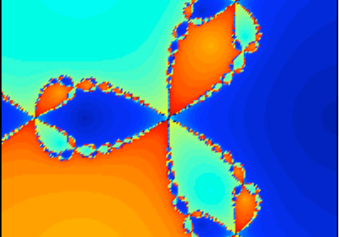Numerical Methods

in Physics & Astrophysics

2020-21

Course Description

This introductory course in numerical analysis covers a wide range of methods and applications in physics and astrophysics.

The first lectures deal with introductory problems, such as solutions of nonlinear equations, stability and convergence and continue with the exact or approximate solutions of linear and non-linear systems of equations.

Considerable time is spend in the numerical solutions of ordinary differential equations, and their applications.

Finally, theoretical and numerical methods for the solution of partial differential equations are discussed in detail.

Special lectures on Finite Element method are delivered by Dr. E. Gaertig.

The course is accompanied with laboratory work. In the labs the students learn to program in C (or C++), python, Matlab,  and they write their own routines to solve problems related to the theoretical part of the course.Related SitesNumerical Analysis

Teaching/exercise hours

Theory     : Tuesday 14:00-15:30

Exercises : Friday    12:00-13:30  (indicative)

ONLY VIA INTERNET

1st LECTURE 3.10.2020

Student hours

Tuesday      : 13:00 - 14:00

Wednesday  : 13:00 - 14:00

Lecture Notes

Finite Element Example Code (by Erich Gaertig)

1. 10. Finite Element Methods (old)

The GATech version (Pablo Laguna)